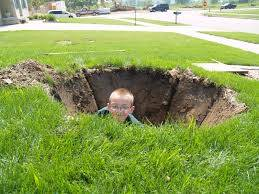# How Much Dirt In A Hole Five Feet Deep, Three Feet Wide, And Four Feet Long?

The answer is zero. As a hole, the area contains no dirt. If it did, it wouldn't be a hole anymore. If you mean how much dirt was displaced in digging the hole in the first place, that's something different.Calculating the volume of an area is simple enough if we have the length, width and depth of the area concerned. The formula is as follows:

Volume = length x width x area.

In this instance, we have:

Volume = (4 feet x 3 feet) x 5 feet.

Volume = 12 feet x 5 feet

Volume of the hole/displaced dirt is therefore 60 feet cubed.

If you were asked the question as a trick question, get your own back by learning a few of your own at you-can-be-funny.com or creatievepuzzels.com. To learn more about volume, try basic-mathematics.com, helpingwithmath.com or watch this YouTube film on how to calculate volume.

thanked the writer.